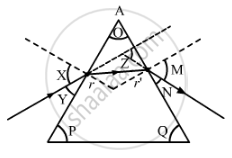# The Path of a Ray of Light Passing Through a Glass Prism - Science

Short Note

The path of a ray of light passing through a glass prism is shown below:In this diagram, the angle of prism, angle of incidence, angle of emergence and angle of deviation, respectively, have been represented by:

(A) O, Y, Z and N,

(B) P, Y, M and Z,

(C) O, X, M and Z,

(D) P, X, Z and N.

#### Solution

O is the angle of prism.

Angle of incidence is the angle made by the incident ray with the normal to the surface of the prism. Here, X represents the angle of incidence.

Angle of emergence is the angle made by the emerging ray with the normal to the surface of the prism. Here, Mrepresents the angle of emergence.

Angle of deviation is the angle made by the emerging ray with the incident ray. Here, Z represents the angle of deviation.

Hence, the correct option is C.

Concept: Dispersion of Light Through Prism and Formation of Spectrum
Is there an error in this question or solution?